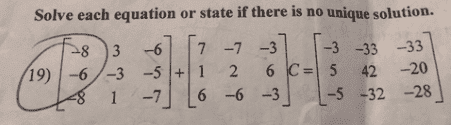# Matrix help — Solve this matrix equation

Poster has been reminded to show their work on the problem in order to get our tutorial help

## Homework Statement## The Attempt at a Solution

I know I would have to do something with my calculator and I tried to solve like solving an equation for C, but not sure. I put all the matrices in my calculator. I then subtracted the first matrix to the other side then multiplied by the inverse of the second. That got my out a 3x3 matrix, but not sure if that means that is the solution.

#### Attachments

Last edited:

Homework Helper
Gold Member
From the looks of it, ## C ## must be a 3x3 matrix.

LCKurtz
Homework Helper
Gold Member

## Homework Statement

View attachment 226616

## The Attempt at a Solution

I know I would have to do something with my calculator and I tried to solve like solving an equation for C, but not sure. I put all the matrices in my calculator. I then subtracted the first matrix to the other side then multiplied by the inverse of the second. That got my out a 3x3 matrix, but not sure if that means that is the solution.
That sounds like the correct thing to do. If you are unsure, put your answer in and see if it works.

•Entr0pic and scottdave
Mark44
Mentor
If you are unsure, put your answer in and see if it works.
Substituting your answer in the original equation is a good idea in any case. As you progress in mathematics, some of the calculations get very involved, and there are lots opportunities to make small errors. Once you reach a solution, you can confirm that your answer is correct by substituting it in the problem. If you end up with two equal expressions, that's a strong indication that your solution is correct.

•scottdave
scottdave